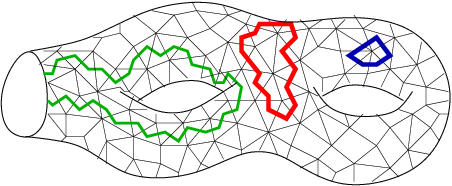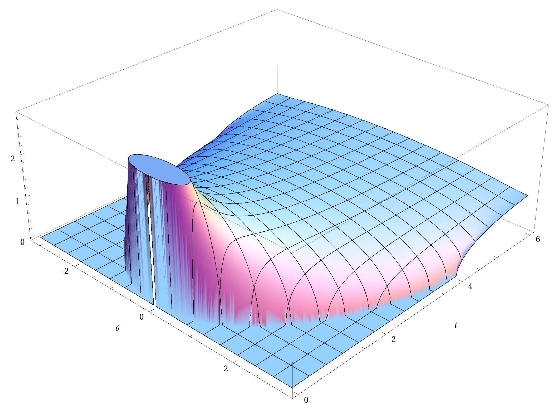# GranMa 2011

## Institut Henri Poincaré, Paris

General

Announcement

Participants

Practical details

Registration

Schedule

Camille Male, ENS Lyon:

## The strong asymptotic freeness of random and deterministic matrices

In this talk, I will present a strengthened version of Voiculescu's asymptotic freeness theorem for random matrices. Let $\mathbf X_N= (X_1^{(N)} , \dots , X_p^{(N)})$ be a family of $N$ by $N$ independent, normalized random matrices from the Gaussian Unitary Ensemble and $\mathbf U_N = (U_1^{(N)} , \dots , U_q^{(N)})$ be a family of $N$ by $N$ independent Haar matrices on the unitary group. We characterize the families of matrices $\mathbf Y_N =(Y_1^{(N)} , \dots , Y_q^{(N)})$, possibly random but independent of $(\mathbf X_N, \mathbf U_N)$, for which the operator norm of $P(\mathbf X_N, \mathbf U_N, \mathbf Y_N, \mathbf Y_N^*)$ converges almost surely for all polynomials $P$. As a consequence of this result, we get the well known Bai and Silverstein's "no eigenvalues outside the support of limiting empirical spectral distribution"'s phenomena for a large class of hermitian random matrices.